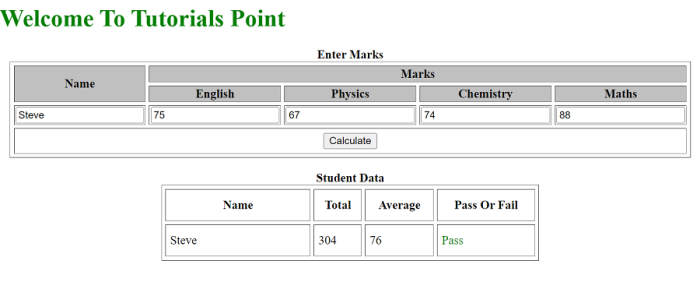# Creating a Dynamic Report Card using HTML, CSS, and JavaScript

In this article, we are going to build a dynamic report using the inputs entered by the user. The user will enter the marks (in this case) and we will be populating these marks to calculate the final percentage of the student.

We have also implemented the fail/pass status that will render whether a student has passed or failed based upon the entries done by the user. We will be using HTML, CSS, and JavaScript for the implementation.

## Steps

• We will be creating an HTML template with rows and columns for name and subject scores. These subject scores will be further divided into 4 columns that describe the 4 subject names to be displayed.

• There will be another student data table with the following columns: Name, Total, Average Percentage, and Pass/Fail.

• For every entry made towards the data, one row will be added to the score table that will show the total student’s score, average score, and pass or fail status.

• The pass/fail status will depend upon the student’s average score. If the average score >= 33, it is pass else fail.

• Any other data will be stored in the table.

## Example 1

In this example, we are fetching the marks from the user and then calculating the total and the average score. Based on this average score we are fetching whether the student has passed or failed.

# index.html

<!DOCTYPE html>
<html>
<body>
<h1 style="color: green;">
Welcome To Tutorials Point
</h1>
<center>
<table border="1" cellspacing="5" bgcolor="white">
<caption><b>Enter Marks</b></caption>
<tr style="background: silver;">
<th rowspan="2">Name</th>
<th colspan="4">Marks</th>
</tr>
<tr style="background: silver;">
<th>English</th>
<th>Physics</th>
<th>Chemistry</th>
<th>Maths</th>
</tr>
<tr>
<td><input type="text" id="name"></td>
<td><input type="text" id="english"></td>
<td><input type="text" id="physics"></td>
<td><input type="text" id="chem"></td>
<td><input type="text" id="maths"></td>
</tr>
<tr>
<th colspan="5" height="30">
<input type="submit" value="Calculate" onclick="Sub()"></th>
</tr>
</table>
<br>
<table border="1" cellspacing="5" bgcolor="white"
<caption><b>Student Data</b></caption>
<tr>
<th width="180">Name</th>
<th>Total</th>
<th>Average</th>
<th>Pass Or Fail</th>
</tr>
</table>
</center>
<script type="text/javascript">
function Sub(){
var n, k, r, e, v, sum, avg;
n=(document.getElementById('name').value);
eng=parseFloat(document.getElementById('english').value);
phy=parseFloat(document.getElementById('physics').value);
chem=parseFloat(document.getElementById('chem').value);
maths=parseFloat(document.getElementById('maths').value);
// Calculating the Total Marks
sum=eng+phy+chem+maths;
avg=sum/4;
// Displaying the Student Data
var newTable = document.getElementById('TableScore');
var row = newTable.insertRow(-1);
var cell1 = row.insertCell(0);
var cell2 = row.insertCell(0);
var cell3 = row.insertCell(0);
var cell4 = row.insertCell(0);
cell4.innerHTML= n;
cell3.innerHTML=sum;
cell2.innerHTML = avg;
if(avg>=33){
cell1.innerHTML="<font color=green>Pass</font>";
} else {
cell1.innerHTML="<font color=red>Fail</font>";
}
}
</script>
</body>
</html>

## Output

The above program on successful execution will produce the dynamic report card. In the report card you can add Name and Marks. In Marks you can enter marks for English, Physics, Chemistry and Maths. After entering the marks click the “Calculate” button. It will generate a report cart with Total, Average marks or Pass or Fail status. See a sample screenshot below